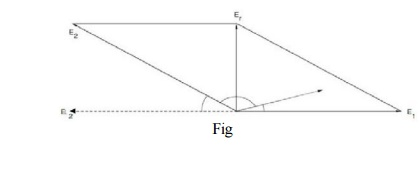Home | | Electrical Machines II | Synchronizing Current

# Synchronizing Current

If two alternators generating exactly the same emf are perfectly synchronized, there is no resultant emf acting on the local circuit consisting of their two armatures connected in parallel.

Synchronizing Current:

If two alternators generating exactly the same emf are perfectly synchronized, there is no resultant emf acting on the local circuit consisting of their two armatures connected in parallel. No current circulates between the two and no power is transferred from one to the other. Under this condition emf of alternator 1, i.e. E1 is equal to and in phase opposition to emf of alternator 2, i.e. E2 as shown in the Figure .There is, apparently, no force tending to keep them in synchronism, but as soon as the conditions are disturbed a synchronizing force is developed, tending to keep the whole system stable. Suppose one alternator falls behind a little in phase by an angle . The two alternator emfs now produce a resultant voltage and this acts on the local circuit consisting of the two armature windings and the joining connections. In alternators, the synchronous reactance is large compared with the resistance, so that the resultant circulating current Is is very nearly in quadrature with the resultant emf Er acting on the circuit. Figure represents a single phase case, where E1 and E2 represent the two induced emfs, the latter having fallen back slightly in phase. The resultant emf, Er, is almost in quadrature with both the emfs, and gives rise to a current, Is, lagging behind Er by an angle approximating to a right angle. It is, thus, seen that E1 and Is are almost in phase. The first alternator is generating a power E1 Is cos  1, which is positive, while the second one is generating a power E2 Is cosФ2, which is negative, since cosФ2 is negative. In other words, the first alternator is supplying the second with power, the difference between the two amounts of power represents the copper losses occasioned by the current Is flowing through the circuit which possesses resistance. This power output of the first alternator tends to retard it, while the power input to the second one tends to accelerate it till such a time that E1 and E2 are again in phase opposition and the machines once again work in perfect synchronism. So, the action helps to keep both machines in stable synchronism. The current, Is, is called the synchronizing current.Study Material, Lecturing Notes, Assignment, Reference, Wiki description explanation, brief detail
Electrical machines : Synchronous Generator : Synchronizing Current |